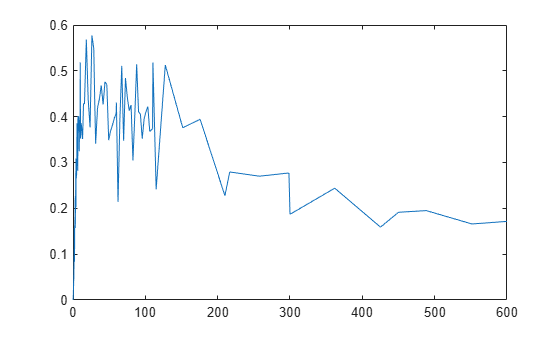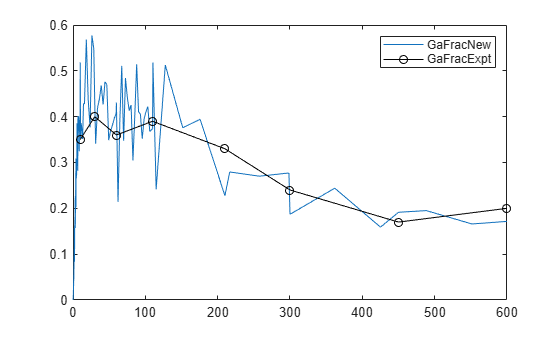# random

Simulate SimBiology model, adding variations by sampling error model

## Syntax

``````[ynew,parameterEstimates] = random(resultsObj)``````
``````[ynew,parameterEstimates] = random(resultsObj,data,dosing)``````
``````[ynew,parameterEstimates]= random(resultsObj,data,dosing,'Variants',v)``````

## Description

example

``````[ynew,parameterEstimates] = random(resultsObj)``` returns simulation results `ynew` with added noise using the error model information specified by the `resultsObj.ErrorModelInfo` property and estimated parameter values `parameterEstimates`.```

example

``````[ynew,parameterEstimates] = random(resultsObj,data,dosing)``` uses the specified `data` and `dosing` information.```
``````[ynew,parameterEstimates]= random(resultsObj,data,dosing,'Variants',v)``` also applies the specified variants to each simulation. NoteThe noise is only added to states that are responses which are the states included in the `responseMap` input argument when you called `sbiofit` or the ResponseMap property of `fitproblem`. If there is a separate error model for each response, the noise is added to each response separately using the corresponding error model. ```

## Examples

collapse all

This example uses the yeast heterotrimeric G protein model and experimental data reported by . For details about the model, see the Background section in Parameter Scanning, Parameter Estimation, and Sensitivity Analysis in the Yeast Heterotrimeric G Protein Cycle.

`sbioloadproject gprotein`

Store the experimental data containing the time course for the fraction of active G protein.

```time = [10 30 60 110 210 300 450 600]'; GaFracExpt = [0.35 0.4 0.36 0.39 0.33 0.24 0.17 0.2]';```

Create a groupedData object based on the experimental data.

```tbl = table(time,GaFracExpt); grpData = groupedData(tbl);```

Map the appropriate model component to the experimental data. In other words, indicate which species in the model corresponds to which response variable in the data. In this example, map the model parameter `GaFrac` to the experimental data variable `GaFracExpt` from `grpData`.

`responseMap = 'GaFrac = GaFracExpt';`

Use an `estimatedInfo` object to define the model parameter `kGd` as a parameter to be estimated.

`estimatedParam = estimatedInfo('kGd');`

Perform the parameter estimation. Use the name-value argument `ErrorModel` to specify the error model that adds error to simulation data.

`fitResult = sbiofit(m1,grpData,responseMap,estimatedParam,ErrorModel="proportional");`

View the estimated parameter value of `kGd`.

`fitResult.ParameterEstimates`
```ans=1×3 table Name Estimate StandardError _______ ________ _____________ {'kGd'} 0.10877 0.001397 ```

Use the `random` method to retrieve the simulation data with added noise using the proportional error model which was specified by `sbiofit`. Note that the noise is added only to the response state, that is the `GaFrac` parameter.

`[ynew,paramEstim] = random(fitResult);`

Select the simulation data for the `GaFrac` parameter.

`GaFracNew = select(ynew,{'Name','GaFrac'});`

Plot the simulation results.

```plot(GaFracNew.Time,GaFracNew.Data) hold on```Plot the experimental data to compare it with the simulated data.

```plot(time,GaFracExpt,'Color','k','Marker','o') legend('GaFracNew','GaFracExpt')```## Input Arguments

collapse all

Estimation results, specified as an `OptimResults object` or `NLINResults object`, which contains estimation results returned by `sbiofit`. It must be a scalar object.

Grouped data or output times, specified as a ```groupedData object```, vector, or cell array of vectors of output times.

If it is a vector of time points, `random` simulates the model with new time points using the parameter estimates from the results object `resultsObj`.

If it is a cell array of vectors of time points, `random` simulates the model n times using the output times from each time vector, where n is the length of `data`.

If it is a `groupedData` object, it must have an independent variable such as Time. It must also have a group variable if the training data used for fitting has such variable. You can use a `groupedData` object to query different combinations of categories if the `resultsObj` contains parameter estimates for each category. `random` simulates the model for each group with the specified categories. For instance, suppose you have a set of parameter estimates for sex category (males versus females), and age category (young versus old) in your training data. You can use `random` to simulate the responses of an old male (or any other combination) although such patient may not exist in the training data.

If the `resultsObj` is from estimating category-specific parameters, `data` must be a `groupedData` object.

Note

If `UnitConversion` is turned on for the underlying SimBiology model that was used for fitting and `data` is a `groupedData` object, `data` must specify valid variable units via `data.Properties.VariableUnits` property. If it is a numeric vector or cell array of vectors of time points, `random` uses the model’s `TimeUnits`.

Dosing information, specified as empty `[]` or `{}`, 2-D matrix or cell vector of SimBiology dose objects (`ScheduleDose object` or `RepeatDose object`).

If `dosing` is empty, no doses are applied during simulation, even if the model has active doses.

For a matrix of dose objects, it must have a single row or one row per group in the input data. If it has a single row, the same doses are applied to all groups during simulation. If it has multiple rows, each row is applied to a separate group, in the same order as the groups appear in the input data. Multiple columns are allowed so that you can apply multiple dose objects to each group.

For a cell vector of doses, it must have one element or one element per group in the input data. Each element must be `[]` or a vector of doses. Each element of the cell is applied to a separate group, in the same order as the groups appear in the input data.

In addition to manually constructing dose objects using `sbiodose`, if the input `groupedData` object has dosing information, you can use the `createDoses` method to construct doses.

Dose objects of the `dosing` input must be consistent with the original dosing data used with `sbiofit`. The objects must have the same values for dose properties (such as `TargetName`) or must be parameterized in the same way as the original dosing data. For instance, suppose that the original dosing matrix has two columns of doses, where the doses in the first column target species x and those in the second column target species y. Then `dosing` must have doses in the first column targeting species x and those in the second column targeting species y. A parameterized dose example is as follows. Suppose that the `Amount` property of a dose used in the original `sbiofit` call is parameterized to a model-scoped parameter `'A'`. All doses for the corresponding group (column) in the `dosing` matrix input must have the `Amount` property parameterized to `'A'`.

The number of rows in the `dosing` matrix or number of elements in the `dosing` cell vector and the number of groups or output time vectors in `data` determine the total number of simulation results in the output `ynew`. For details, see the table in the `ynew` argument description.

Note

If `UnitConversion` is turned on for the underlying SimBiology® model that was used for fitting, `dosing` must specify valid amount and time units.

Variants to apply, specified as an empty array (`[]`, `{}`), 2-D matrix or cell vector of variant objects.

If you do not specify this argument, the function has the following behavior depending on whether the second input argument (`data`) is specified also or not.

• If `data` is not specified, the function applies the group-specific variants from the original call to `sbiofit`.

• If `data` is a vector or cell array of output times, the function does not apply the group-specific variants.

• If `data` is a `groupedData` object, the function applies variants only to groups whose group identifier matches a group identifier in the original training data that was used in the call to `sbiofit`.

Note

• The baseline variants that were specified by the variants positional input argument in the original call to `sbiofit` are always applied to the model, and they are applied before any group-specific variants.

• If there are no baseline variants, that is, you did not specify the `variants` input when calling `sbiofit`, the `random` function still applies the model active variants if there are any.

If the argument value is `[]` or `{}`, the function applies no group-specific variants.

If it is a matrix of variants, it must have either one row or one row per group. Each row is applied to a separate group, in the same order as the groups appear in `data` or `dosing`. If it has a single row, the same variants are applied to all groups during simulation. If there are multiple columns, the variants are applied in order from the first column to the last.

If it is a cell vector of variant objects, the number of cells must be one or must match the number of groups in the input data. Each element must be `[]` or a vector of variants. If there is a single cell containing a vector of variants, they are applied to all simulations. If there are multiple cells, the variants in the ith cell are applied to the simulation of the ith group.

The function defines the number of groups by examining the `data`, and `dosing` input arguments.

• `data` can have `1` or N groups.

• If `data` and `dosing` arguments are not specified, then the default data and dosing are determined as follows:

• For unpooled fits, they are the data and dosing for the single group associated with that fit results.

• For all other fits, they are the entire set of data and dosing associated with the call to `sbiofit`.

## Output Arguments

collapse all

Simulation results, returned as a vector of `SimData` objects. The states reported in `ynew` are the states that were included in the `responseMap` input argument of `sbiofit` as well as any other states listed in the `StatesToLog` property of the runtime options (`RuntimeOptions`) of the SimBiology model.

The total number of simulation results in `ynew` depends on the number of groups or output time vectors in `data` and the number of rows in the `dosing` matrix.

Number of groups or output time vectors in `data`Number of rows in the `dosing` matrixSimulation results

`1`

`0`, that is, `dosing` is empty `[]`

The total number of `SimData` objects in `ynew` is 1.

No doses are applied during simulation.

`1`

`1`

The total number of `SimData` objects in `ynew` is 1.

The given row of doses is applied during the simulation.

`1`

N

The total number of `SimData` objects in `ynew` is N.

Each row of `dosing` is applied to each simulation.

N

`0`, that is, `dosing` is empty `[]`

The total number of `SimData` objects in `ynew` is N.

No doses are applied during simulation.

N

`1`

The total number of `SimData` objects in `ynew` is N.

The same row of doses is applied to each simulation.

NN

The total number of `SimData` objects in `ynew` is N.

Each row of `dosing` is applied to a separate group, in the same order that groups appear in `data`.

MNThe function throws an error when MN.

Estimated parameter values, returned as a table. This is identical to `resultsObj.ParameterEstimates` property.

 Yi, T-M., Kitano, H., and Simon, M. (2003). A quantitative characterization of the yeast heterotrimeric G protein cycle. PNAS. 100, 10764–10769.